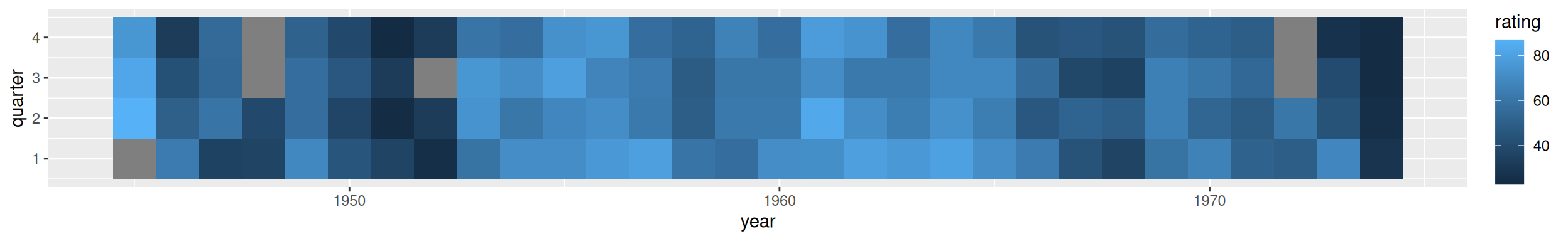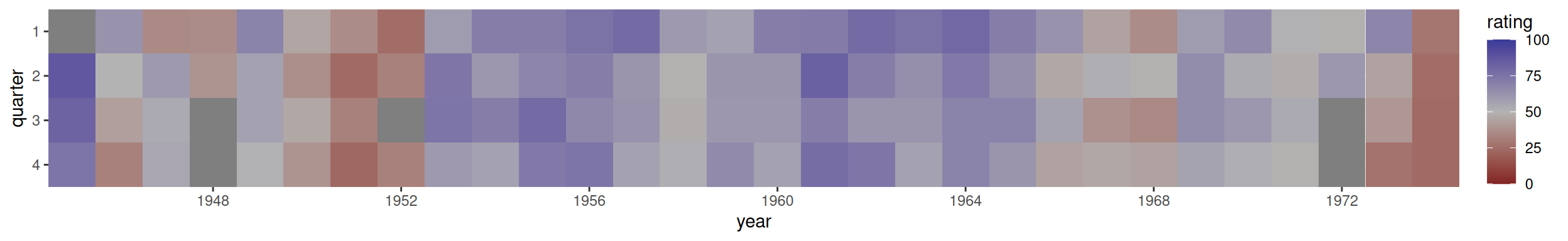## 13.6 Creating a Heat Map

### 13.6.1 Problem

You want to make a heat map.

### 13.6.2 Solution

Use `geom_tile()` or `geom_raster()` and map a continuous variable to `fill`. We’ll use the `presidents` data set, which is a time series object rather than a data frame:

``````presidents
#>      Qtr1 Qtr2 Qtr3 Qtr4
#> 1945   NA   87   82   75
#> 1946   63   50   43   32
#> 1947   35   60   54   55
#>  ...
#> 1972   49   61   NA   NA
#> 1973   68   44   40   27
#> 1974   28   25   24   24
str(presidents)
#>  Time-Series [1:120] from 1945 to 1975: NA 87 82 75 63 50 43 32 35 60 ...``````

We’ll first convert it to a format that is usable by ggplot: a data frame with columns that are numeric:

``````pres_rating <- data.frame(
rating = as.numeric(presidents),
year = as.numeric(floor(time(presidents))),
quarter = as.numeric(cycle(presidents))
)
pres_rating
#>     rating year quarter
#> 1       NA 1945       1
#> 2       87 1945       2
#> 3       82 1945       3
#>  ...<114 more rows>...
#> 118     25 1974       2
#> 119     24 1974       3
#> 120     24 1974       4``````

Now we can make the plot using `geom_tile()` or `geom_raster()` (Figure 13.12). Simply map one variable to `x`, one to `y`, and one to `fill`:

``````# Base plot
p <- ggplot(pres_rating, aes(x = year, y = quarter, fill = rating))

# Using geom_tile()
p + geom_tile()

# Using geom_raster() - looks the same, but a little more efficient
p + geom_raster()``````Figure 13.12: A heat map-the grey squares represent `NA`s in the data

Note

The results with `geom_tile()` and `geom_raster()` should look the same, but in practice they might appear different. See Recipe 6.12 for more information about this issue.

### 13.6.3 Discussion

To better convey useful information, you may want to customize the appearance of the heat map. With this example, we’ll reverse the y-axis so that it progresses from top to bottom, and we’ll add tick marks every four years along the x-axis, to correspond with each presidential term. For the x and y scales, we remove the padding by using `expand=c(0, 0)`. We’ll also change the color scale using `scale_fill_gradient2()`, which lets you specify a midpoint color and the two colors at the low and high ends (Figure 13.13):

``````p +
geom_tile() +
scale_x_continuous(breaks = seq(1940, 1976, by = 4), expand = c(0, 0)) +
scale_y_reverse(expand = c(0, 0)) +
scale_fill_gradient2(midpoint = 50, mid = "grey70", limits = c(0, 100))``````Figure 13.13: A heat map with customized appearance